# NCERT Solutions For Class 10 Maths Chapter 12 Exercise 12.3

Go back to  'Areas Related to Circles'

## Chapter 12 Ex.12.3 Question 1

Find the area of the shaded region in the figure, if $$PQ = 24\,\rm{cm},$$ $$PR = 7\rm\,{cm}$$ and $$O$$ is the centre of the circle.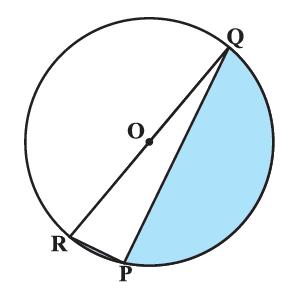### Solution

What is known?

$$PQ = 24\, {\rm{cm}}, PR = 7\,{\rm{cm}},\, O$$ is the center the circle.

Use \begin{align}\pi = \frac{{22}}{7}\end{align}

What is unknown?

Area of the shaded region in figure.

Reasoning:

(i) Visually it’s clear that

Area of the shaded region $$=$$ Area of semicircle $$RPQ \,-$$  Areaof $$\Delta RPQ$$

\begin{align}= {\frac{1}{2}\pi = {{(OR)}^2} - {\text{Area of }}\Delta \rm{RPQ}}\end{align}

Since we don’t know $$RQ$$ (diameter) $$OR$$ and $$PQ$$ (radii of circle), we are unable to find either area of semicircle and we don’t know $$RQ$$ so we can’t find area of $$\Delta RPQ$$ using heron’s formula (since either $$2$$ sides are known) or by\begin{align} \frac{1}{2} \times {\text{base}} \times {\text{height}}\end{align} as we have only $$2$$ sides and that their respective heights.

So, we need to find $$RQ.$$

(ii) Using the knowledge:

Angle subtended by an arc at any point on the circle is half of the angle subtended by it at the center.

\begin{align} \therefore \angle ROQ &= {{180}^\circ } \\ \therefore \angle RPQ &= \frac{1}{2} \times {{180}^\circ = {{90}^\circ }} \end{align}

($$\rm{OR}$$) angle in a semicircle $$= {90^ \circ }$$

We get $$\Delta RQP$$ is a right angle triangle.

Using Pythagoras theorem

\begin{align} RQ^2 &= PR^2 + PQ^2 \end{align}

We get \begin{align} RQ &= \sqrt PR^2 + {PQ^2} \\\therefore \text{radius} &= \frac{1}{2}\,RQ.\end{align}

We can find the area of semicircle.

And since $$\angle RPQ = {90^ \circ }$$

$$\therefore\; RP$$ is the height for $$PQ$$ or vice versa

So using the formula.

Area of \begin{align} \Delta = \frac{1}{2} \times {\text{base}} \times {\text{height}}\end{align}

Area of \begin{align} \Delta \,RPQ = \frac{1}{2} \times PQ \times RP \end{align}

Steps:

$$PQ = 24\,{\rm{cm}}\,\,, PR = 7\,\rm{cm}$$

Hence the angle in a semicircle is a right angle

$$\therefore \;LRPQ = {90^\circ }$$

$$\Rightarrow \Delta {RQP}$$ is a right angled triangle.

$$\therefore \;$$ Using Pythagoras theorem.

\begin{align}{P{Q^2}} &= {P{R^2}} + {P{Q^2}}\\{RQ }&= \sqrt {{7^2} + {{24}^2}} \\ &= \sqrt {49 + 576} \\ &= \sqrt {625} \\ &= 25\,{\rm{cm}}\end{align}

$$\therefore\;$$Radius ($$r$$)  \begin{align}= \frac{{25}}{2}{\text{cm}}\end{align}

Area of shaded region$$=$$Area of semicircle $$RPQ\; –$$ Area of $$\Delta RQP$$

\begin{align}&= \frac{1}{2} \times \pi {{\text{r}}^{\text{2}}} - \frac{{\text{1}}}{{\text{2}}} \times {{PQ}} \times {RP}\\ &= \frac{1}{2} \times \left[ {\frac{{22}}{7} \times \frac{{25}}{2} \times \frac{{25}}{2} - 24 \times 7} \right]\\ &= \frac{1}{2} \times \left[ {\frac{{6875}}{{14}} - 168} \right]\\ &= \frac{1}{2} \times \left[ {\frac{{6875 - 2352}}{{14}}} \right] = \frac{1}{2} \times \frac{{4523}}{{14}}\\ &= \frac{{4523}}{{28}}\,{\text{cm}^2}\\& = 161.535\,\,{\text{cm}^2}\\ &= 161.54\,\,{\text{cm}^2}\,\left( {{\text{approximately}}} \right)\end{align}

## Chapter 12 Ex.12.3 Question 2

Find the area of the shaded region in the given figure, if radii of the two concentric circles with center $$O$$ are $$\text{7 cm}$$ and $$\text{14 cm}$$ respectively and $$\,\angle AOC = {40^ \circ }.$$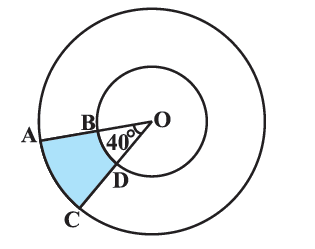### Solution

What is known?

Radius of $$2$$ concentric circles with centre $$O$$ are $$\text{7 cm}$$ and $$\text{14 cm}$$ and$$\,\angle AOC = {40^ \circ }.$$

What is unknown?

Reasoning:

In a circle with radius r and angle at the center with degree measure $$\theta$$

Area of the sector\begin{align} = \frac{{\rm{\theta }}}{{{{360}^{\rm{o}}}}} \times \pi {r^2}\end{align}

Area of the shaded region can be calculated by subtracting the area of the sector of smaller circle from the area of the sector of the larger circle.

Area of shaded region $$ABDC \,=$$  Area of sector $$ACO\, –$$ Area of sector $$BDO$$

\begin{align}\frac{\theta }{{{{360}^\circ }}} \times \pi {(OA)^2} - \frac{\theta }{{{{360}^\circ }}} \times \pi {(OB)^2}.\end{align}

(Using Area of sector of angle $$\theta = \frac{\theta }{{{{360}^\circ }}} \times \pi {r^2}$$ where r is radius of the circle)

\begin{align}\\&={\frac{{40}}{{{{360}^\circ}}} \times \pi \left( {O{A^2} - {OB^2}} \right)}\\&= {\frac{1}{2} \times \pi {(OA + OB)}(OA - OB)}\\\end{align}

(Using algebraic identity \begin{align}{a^2} - {b^2} = (a + b)(a - b)\end{align} to simplify calculations.)

Steps:

Radius of the larger circle,\begin{align}R = OA = 14cm\end{align}

Radius of the smaller circle,\begin{align}r = OB = 7cm\end{align}

Angle at the center,\begin{align}{\rm{\theta }} = {40^{\rm{\circ}}}\end{align}

Area of shaded region $$ABDC =$$  Area of sector $$ACO\; –$$Area of sector $$BDO$$

\begin{align} &= \frac{\theta }{{{{360}^\circ }}}\!\times\! \pi {(OA)^2} \!-\! \frac{\theta }{{{{360}^\circ }}} \!\times\! \pi {(OB)^2}\\& = \frac{{40}}{{{{360}^\circ }}} \times \pi \left( {{{14}^2} - {7^2}} \right)\\ &= \frac{1}{9} \times \frac{{22}}{7} \times (14 + 7)(14 - 7)\\ &= \frac{{22 \times 21 \times 7}}{{9 \times 7}}\,\,{\text{c}}{{\text{m}}^2}\\& = \frac{{22 \times 7}}{3}\\ &= \frac{{154}}{3}\,{\text{c}}{{\text{m}}^2}\end{align}

## Chapter 12 Ex.12.3 Question 3

Find the area of the shaded region in the given figure, if $$ABCD$$ is a square of side $$\text{14 cm}$$ and $$APD$$ and $$BPC$$ are semicircles.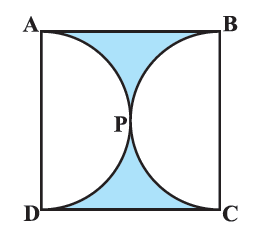### Solution

What is known?

$$ABCD$$ is a square of side ($$l$$) $$\text{14 cm.}$$ $$APD$$ and $$BPC$$ are semicircles.

What is unknown?

Reasoning:

(i) From figure it is clear that diameter of both semicircles $$=$$ side of square $$=\text{14 cm}$$

$$\therefore \;$$ Radius of each semicircle \begin{align} (r) = \frac{{14}}{2} = 7{\text{cm}}\end{align}

(ii) Visually, it is clear

Area of shaded region = Area of square $$ABCD\, -$$ (Area of semicircle $$APD\, +$$ Area of semicircle $$BPC$$)

\begin{align} &= {\left( \rm{side} \right)^2} - \left( {\frac{{\pi {r^2}}}{2} + \frac{{\pi {r^2}}}{2}} \right)\\ &= {\left( \rm{side} \right)^2} - \pi {r^2}\end{align}

Steps:

Since semicircles $$APD$$ and $$BPC$$ are drawn using sides $$AD$$ and $$BC$$ respectively as diameter.

$$\therefore \;$$Diameter of each semicircle $$=\,\text{14 cm}$$

Radius of each semicircle \begin{align}(r) = \frac{{14}}{2} = 7{\text{cm}}\end{align}

Area of shaded region $$=$$ Area of square $$ABCD \,-$$ (Area of semicircle $$APD \,+$$Area of semicircle $$BPC$$)

\begin{align}&{ = {{({\text{side}})}^2} - \left( {\frac{1}{2}\pi {r^2} + \frac{1}{2}\pi {r^2}} \right)}\\&{ = {{14}^2} - \pi \times {{(7)}^2}}\\ &{ = 196 - \frac{{22}}{7} \times 7 \times 7}\\&{ = 196 - 154}\\&{ = 42\,{\text{c}}{{\text{m}}^2}}\end{align}

## Chapter 12 Ex.12.3 Question 4

Find the area of the shaded region in the given figure, where a circular arc of radius $$\text{6 cm}$$ has been drawn with vertex $$O$$ of an equilateral triangle $$OAB$$ of side $$\text{12 cm}$$ as centre.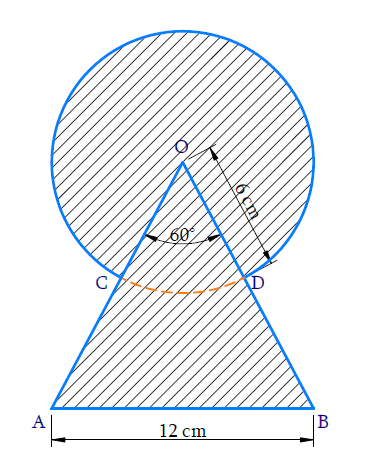### Solution

What is known?

A circular arc of radius $$\text{= 6 cm}$$ is drawn with vertex $$O$$ of an equilateral $$\Delta {OAB}$$ of side $$\text{12 cm}$$ as centre.

What is unknown?

Reasoning:

From the figure it is clear that the shaded region has an overlap region of area of a sector of angle $${60^ \circ }$$ (since each angle of an equilateral $$\Delta$$ is of measure $${60^ \circ }$$ area of a circle with radius $$\text{6 cm}$$ and area of triangle $$OAB$$ with side $$\text{12 cm.}$$

$$\therefore\;$$Area of shaded region $$=$$ Area of circle with radius $$\text{6 cm}\; +$$ Area of $$\Delta {OAB}$$ - Area of sector of angle $${60^ \circ }$$

\begin{align} = \pi {(6)^2} + \frac{{\sqrt {3{{(12)}^2}} }}{4} - \frac{{{{60}^\circ}}}{{{{360}^\circ}}} \times \pi {(6)^2}\end{align}

Using formula, Area of an equilateral \begin{align} \Delta = \frac{{\sqrt 3 }}{4}{({\mathop{\text sid}\nolimits} e)^2}\end{align}

Area of the sector of angle \begin{align} \theta = \frac{\theta }{{{{360}^\circ }}} \times \pi {r^2} \end{align}

Where $$r$$ is the radius of the circle

Steps:

Radius of circle $$(r) =\text{ 6 cm}$$

Side of equilateral $$\Delta {OAB(s)} = 12\,{\text{cm}}$$

We know each interior angle of equilateral $$\Delta = {60^\circ }$$

Since they overlap, part of area of a sector $$OCD$$ is in area of the circle and triangle.

$$\therefore \;$$ Area of shaded region = Area of circle $$+$$ Area of $$\Delta {OAB}\,-$$Area of sector $$OCD$$ of angle $$60^\circ$$

\begin{align} &= \pi {\left( 6 \right)^2} + \frac{{\sqrt 3 }}{4}{\left( {12} \right)^2} - \frac{{60}}{{360}} \times \pi {\left( 6 \right)^2}\\ &= 36\pi + 36\sqrt 3 - \frac{{36}}{6}\pi \\ &= 36\left( {\pi + \sqrt 3 - \frac{\pi }{6}} \right)\\ &= 36\left( {\sqrt 3 + \frac{{5\pi }}{6}} \right)\\ &= 36\sqrt 3 + 30\pi \\ &= 36\sqrt 3 + 30 \times \frac{{22}}{7}\\ &= 36\sqrt 3 + \frac{{660}}{7}\,{\text{c}}{{\text{m}}^2}\end{align}

## Chapter 12 Ex.12.3 Question 5

From each corner of a square of side $$\text{4 cm}$$ a quadrant of a circle of radius $$\text{1 cm}$$ is cut and also a circle of diameter $$\text{2 cm}$$ is cut as shown in the given figure. Find the area of the remaining portion of the square.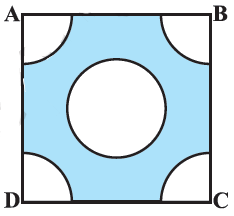### Solution

What is the known?

From each corner of a square of side $$\text{= 4 cm}$$ a quadrant of a circle of radius $$\text{1 cm}$$ is cut and also a circle of diameter $$\text{2 cm.}$$

What is the unknown?

Area of remaining portion of the square.

Reasoning:

(i) Since diameter of circle which is cut out $$\text{= 2 cm}$$

$$\therefore\;$$ Radius of this circle $$(r) =\text{ 1 cm}$$

Area of portions cut out of square $$=$$  Area of the circle $$+$$ 4$$\times$$(Area of each quadrant)

\begin{align}&= \pi {r^2} + 4\left( {\frac{{{{90}^ \circ }}}{{{{360}^ \circ }}} \times \pi {r^2}} \right)\\ &= { \pi {r^2} + 4 \times \frac{{\pi {r^2}}}{4}}\\ &= {\pi {r^2} + \pi {r^2}}\\ &= 2\pi {r^2}\end{align}

(iii) From the figure it is clear that

Area of remaining portion of the square $$=\;$$Area of square $$-$$ Area of portion cut out of square

\begin{align}= {\left( \rm{side} \right)^2} - 2\pi {r^2}\end{align}

Steps:

Diameter of circle $$\text{= 2 cm}$$

Radius of circle \begin{align} (r) = \frac{{2\,{\text{cm}}}}{2} = \,1\,{\text{cm}} \end{align}

Radius of all quadrants cut out (r)=1cm$$\rm{}(r)=1\,cm$$

Area of the portions cut out of the square $$=$$  Area of a full circle  $$+\, 4 \;\times$$  (Area of each quadrant)

\begin{align}&={\pi {r^2} + 4\left( {\frac{{{{90}^\circ }}}{{{{360}^\circ }}} \times \pi {r^2}} \right)}\\ &={ \pi {{(1)}^2} + 4 \times \frac{{\pi {{(1)}^2}}}{4}}\\ &={\pi + \pi }\\ &={2\pi }\end{align}

Area of remaining portion of the square $$=$$ Area of square $$-$$ Area of portions cut out

\begin{align}&= \rm{}{\left( {side} \right)^2} - 2\pi {r^2}\\&=\rm{} {\left( {4cm} \right)^2} - \frac{{44}}{7}c{m^2}\\&= \rm{}16c{m^2} - \frac{{44}}{7}c{m^2}\\&= \rm{}\frac{{112 - 44}}{7}c{m^2}\\&=\rm{} \frac{{68}}{7}c{m^2} \end{align}

## Chapter 12 Ex.12.3 Question 6

In a circular table cover of radius $$\text{32 cm,}$$ a design is formed leaving an equilateral triangle $$ABC$$ in the middle as shown in the given figure. Find the area of the design.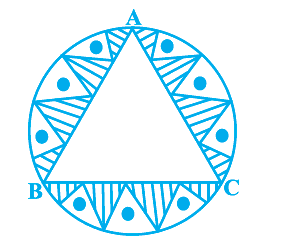### Solution

What is known?

A circular table cover of radius $$\text{= 32 cm,}$$ a design is formed leaving an equilateral triangle $$ABC$$ in the middle as shown in figure.

What is unknown?

The area of the design.

Reasoning: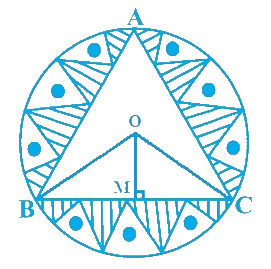Mark $$O$$ as centre of the circle. Join $$BO$$ and $$CO.$$

Since ,we know that equal chords of a circle subtends equal angles at the center and all sides of an equilateral triangle are equal.

$$\therefore \;$$ Each side of $$\Delta$$ will subtend equal angles at centre

\begin{align}\therefore \angle BOC = \frac{{{{360}^\circ }}}{3}\,\, = {120^\circ }\end{align}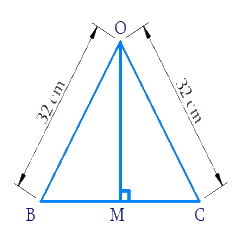Consider$$\Delta BOC$$

Drop a perpendicular from $$OM$$ to $$BC$$

Since we know perpendicular from the center of circle to a chord bisects it

$$\Rightarrow \quad BM = MC$$

$$OB = OC$$ (radii) and $$OM = OM$$(common)

$$\therefore \Delta {OBM} \cong \Delta {OCM}$$ (by SSS congruency)

$$\Rightarrow \angle BOM\, =\,\angle COM$$ (Corresponding Parts of Congruent Triangles are Congrue (CPCTC))

$$\therefore \,2\angle {BOM}=\angle {BOC}={{120}^{\circ }}$$

\begin{align}\angle {BOM}&=\frac{{{120}^{\circ }}}{2}\\&={{60}^{\circ }} \\ \frac{{BM}}{{BO}}&=\sin \,{{60}^{\circ }}\\&=\frac{\sqrt{3}}{2} \\{BM}&=\frac{\sqrt{3}}{2}\times {BO}\\&=\frac{\sqrt{3}}{2}\times 32\\&=16\sqrt{3}\\ \quad {BC}&=2 {BM}\\&=32\sqrt{3}\,cm \\ \end{align}

Using formula of area of equilateral $$\Delta$$

\begin{align} = \frac{{\sqrt 3 }}{4}{({\text{side}})^2}\end{align}

We can find area of $$\Delta {ABC}$$ since a side $$BC$$ of $$\Delta$$ is known.

Visually from figure it’s clear

Area of the design $$=$$  Area of circle $$-$$  Area of $$\Delta ABC$$

\begin{align}= \pi {r^2} - \frac{{\sqrt 3 }}{4}{({BC})^2}\end{align}

This can be solved with ease as both the radius of the circle and $$BC$$ are known.

Steps:Let the center of the circle be $$O.$$ Join $$BO$$ and $$CO.$$

Since equal chords of a circle subtend equal angles as its centre.

$$\therefore \;$$ Sides $$AB, BC$$ and $$AC$$ of $$\Delta ABC$$ will subtend equal angles at the centre of circle

\begin{align}\therefore \angle {BOC} = \frac{{{{360}^\circ }}}{3} = {120^\circ }\end{align}

Draw $${{OM}} \bot {{BC}}$$

In $$\Delta BOM\ {\rm and} \Delta COM$$

$$BO = CO$$ (radii circle)

$$OM = OM$$ (common)

$$BM = CM$$.... (perpendicular drawn from the center of the circle to a chord bisects it)

$$\Delta {BOM} \cong \Delta {COM}$$ (By SSS Congruency)

$$\therefore \;\angle {BOM} = \angle {COM} \dots \dots(2)$$(CPCT)

From figure

\begin{align}\angle {BOM} + \angle {COM} &= \angle {BOC}\\2\angle {BOM} &= \angle {BOC}\\ &= {120^ \circ }\\&\left( {{\text{Using}}\,{\text{ }}\left( 2 \right)} \right)\\\angle {BOM} &= \frac{{{{120}^ \circ }}}{2} = {60^ \circ }\end{align}

In $$\Delta {BOM}$$

\begin{align}\frac{{BM}}{{BO}} &= \sin \,{60^ \circ } = \frac{{\sqrt 3 }}{2}\\\therefore {BM} &\!=\! \frac{{\sqrt 3 }}{2}{BO}\!=\!\frac{{\sqrt 3 }}{2}\!\!\times\!32\!=\!16\sqrt 3 \\{\text{BC}} &= {\text{BM}} + {\text{CM}} = 2{\text{BM}}\\&\qquad({\text{using}}(1))\\\,\,\,\,\,\,\,\, &= 2 \times 16\sqrt 3 \\\,\,\,\,\,\,\,\, &= 32\sqrt 3 \end{align}

Radius of circle $$(r) =\text{ 32 cm}$$

From figure, we observe

Area of design $$=$$ Area of circle $$-$$ Area of $$\Delta {ABC}$$

\begin{align} &={\pi {r^2} - \frac{{\sqrt 3 }}{4}{{(BC)}^2}}\\ &={\pi {{(32)}^2} - \frac{{\sqrt 3 }}{4}{{(32\sqrt 3 )}^2}}\\ &= {\pi {{(32)}^2} - \frac{{\sqrt 3 }}{4}{{(32)}^2} \times 3}\\ &={\frac{{22528}}{7} \times 1024 - 32 \times 24\sqrt 3 }\\ &= {\frac{{22528}}{7} - 768\sqrt 3}\end{align}

Area of Design \begin{align} = \left( {\frac{{22528}}{7} - 768\sqrt 3 } \right)\rm{cm^2}\end{align}

## Chapter 12 Ex.12.3 Question 7

In the given figure, $$ABCD$$ is a square of side $$\text{14 cm.}$$ With Centers $$A, B, C$$ and $$D,$$ four circles are drawn such that each circle touch externally two of the remaining three circles. Find the area of the shaded region.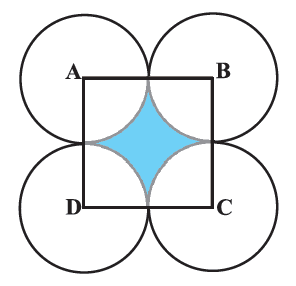### Solution

What is known?

$$ABCD$$ is a square of side $$\text{= 14 cm.}$$

With centers$$A, B, C, D$$ four circles are drawn such that each circle touches externally $$2$$ of the remaining $$3$$ circles.

What is unknown?

Reasoning:

Since the circles are touching each other externally,visually it is clear that

Radius of each circle \begin{align} {r} = \frac{1}{2}\,\, \times \end{align}(side of square)

Also, $$ABCD$$ being a square all angles are of measure $${90^ \circ },\,\,\,$$

Therefore,all sector are equal as they have same radii and angle.

$$\therefore\;$$ Angle of each sector which is part of the square $$\left( {\theta } \right) = {90^ \circ }$$

$$\therefore\;$$$$\text{Area of each sector}$$\begin{align}&= \frac{\theta }{{{{360}^\circ }}} \times \pi {r^2}\\&= \frac{{{{90}^\circ }}}{{{{360}^\circ }}} \times \pi {r^2}\\&= \frac{{\pi {r^2}}}{4}\end{align}

From the figure it is clear that:

Area of shaded region $$=$$  Area of square $$–$$  Area of $$4$$ sectors

\begin{align} &= {\left( {{\text{side}}} \right)^2} - 4 \times {\text{Area of each sector}}\\ &= {\left( {14} \right)^2} - 4 \times \frac{{\pi {r^2}}}{4}\\ &= {\left( {14} \right)^2} - \pi {r^2}\end{align}

Steps:

Area of each of the $$4$$ sectors is equal as each sector subtends an angle of $${90^ \circ }$$ at the centre of a circle with radius $$\text{= 7 cm}$$

$$\therefore$$ $$\text{Area of each sector}$$\begin{align}&= \frac{\theta }{{{{360}^\circ }}} \times \pi {r^2}\\ &= \frac{{{{90}^\circ }}}{{{{360}^\circ }}} \times \pi {(7)^2}\\ &= \frac{1}{4} \times \frac{{22}}{7} \times 7 \times 7\\ &= \frac{{77}}{2}{\text{c}}{{\text{m}}^2}\end{align}

Area of shaded region $$=$$  Area of square $$- \,4 \;\times$$ Area of each sector

\begin{align}&= {(14)^2} - 4 \times \frac{{77}}{2}\\ &= 196 - 154\\ &= 42\,\,{\text{c}}{{\text{m}}^2}\end{align}

## Chapter 12 Ex.12.3 Question 8

Figure depicts a racing track whose left and right ends are semicircular. The distance between the two inner parallel line segments is $$\text{60 m}$$ and they are each $$\text{106 m}$$ long. If the track is $$\text{10 m}$$ wide, find:

(i) The distance around the track along its inner edge

(ii) The area of the track.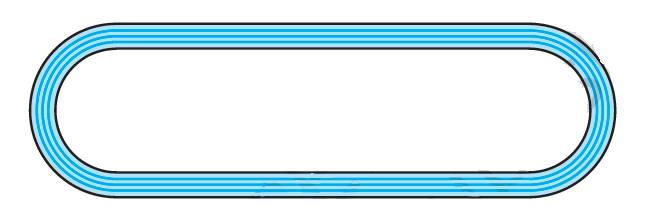### Solution

What is known?

(i) A racing track whose right and left ends are semicircular.

(ii) The distance between the $$2$$ inner parallel line segments is $$\text{60 m}$$ and they are $$\text{106 m}$$ long

(iii) Width of track $$\text{= 10 cm}$$

What is unknown?

(i) The distance around the track along its inner edge.

(ii) Area of the track.

Reasoning: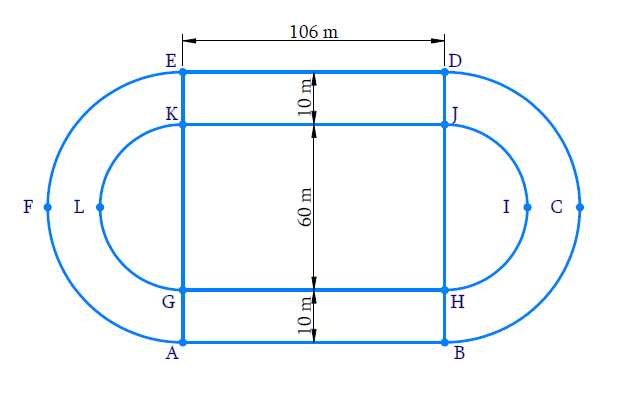(i) Draw a figure along with dimensions to visuals the track properly.

(ii) Visually the distance around the track along its inner edge.

$${ GH + {\rm{arc}}\, HIJ + JK + {\rm{arc}}\, KLG}$$

Where $$GH = JK = \text{106 m}$$

And arc $$HIJ = {\text{arc}}\, KLG =$$ circumference of semicircle with diameter $$\text{60 m.}$$

So, it can be easily found by substituting the required values.

(iii) To find area of track

Visually it’s clear that

Area of the tack = Area of rectangle $$ABHG \;+$$ Area of rectangle $$KJDE \;+$$ (Area of semicircle$$BCD\; –$$ Area of semicircle $$HIJ$$) + (Area of semicircle $$EFA\; –$$ Area of semicircle $$KLG$$)

Radii of semicircles $$HIJ$$ and $$KLG$$\begin{align} {\text{ = }}\frac{{60}}{{\text{2}}}{\text{ = 30 m}}\end{align}

Radii of semicircles $$BCD$$ and $$EFA =\text{ 30 m + 10 m = 40 m}$$

And $$JK = GH =\text{ 106 m}$$

And $$DJ = HB = \text{10 m}$$

Area of the track

=\left[ \begin{align} & GH\times HB+JK\times DJ+ \\ & \left( \frac{1}{2}\pi {{(40)}^{2}}-\frac{1}{2}{{(30)}^{2}} \right)+ \\ & \left( \frac{1}{2}\pi {{(40)}^{2}}-\frac{1}{2}{{(30)}^{2}} \right) \\ \end{align} \right]

Steps:Diameter of semicircle $$HIJ =$$ Diameter of semicircle $$KLG = \rm{60 m}$$

$$\therefore\;$$ their radius \begin{align}\left( {{r_1}} \right) = \frac{{60}}{2} = 30\,{\text{m}}\end{align}

(i) The distance around the track along its inner edge.

\begin{align}& =GH\!+\!\!arc HIJ \!+\!\!JK \!+\!\!arc KLG\\&= 106 + \frac{{2\pi {r_1}}}{2} + 106 + \frac{{2\pi {r_1}}}{2}\\ &= {106 + \pi \times 30 + 106 + \pi \times 30}\\&={ 212 + \frac{{1320}}{7}}\\ &= \frac{{1484 + 1320}}{7}\\ &= \frac{{2804}}{7}\,{\text{m}}\end{align}

(ii) Radius of semicircle $$BCD =$$ Radius of semicircle $$EFA$$

\begin{align}\left( {{r_2}} \right) &= 30\,\rm{m }+ 10m\\ &= 40\,\rm{m }\end{align}

Area of the tack $$=$$ Area of rectangle $$ABHG \,+$$ Area of rectangular $$KJDE \;+$$ (Area of semicircle $$BCD\;-$$ Area of semicircle $$HIJ$$) $$+$$(Area of semicircle $$EFA\; -$$ Area of semicircle $$KLG$$)

\begin{align} & =\left\{ \begin{array} & \left( 106\times 10 \right)+\left( 106\times 10 \right)+ \\ \left[ \frac{1}{2}\pi {{\left( 40 \right)}^{2}}-\frac{1}{2}\pi {{\left( 30 \right)}^{2}} \right]+ \\ \left[ \frac{1}{2}\pi {{\left( 40 \right)}^{2}}-\frac{1}{2}\pi {{\left( 30 \right)}^{2}} \right] \\\end{array} \right\} \\ & =\left\{ \begin{array} & 1060+1060+ \\ \left[ \frac{1}{2}\pi \left( 1600-900 \right) \right]+ \\ \left[ \frac{1}{2}\pi \left( 1600-900 \right) \right] \\ \end{array} \right\} \\ & =\left\{ \begin{array} & 1060+1060+ \\ \frac{\pi }{2}\times 700+\frac{\pi }{2}\times 700 \\ \end{array} \right\} \\ & =2120+700\pi \\ & =2120+700\times \frac{22}{7} \\ & =2120+2200 \\ & =4320{{\text{m}}^{\text{2}}} \\ \end{align}

## Chapter 12 Ex.12.3 Question 9

In Figure, $$AB$$ and $$CD$$ are two diameters of a circle (with center $$O$$) perpendicular to each other and $$OD$$  is the diameter of the smaller circle. If $$OA = \rm{7 cm,}$$ find the area of the shaded region.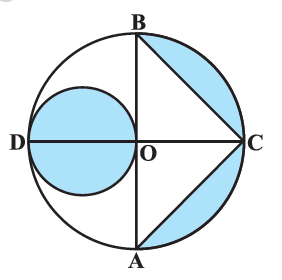### Solution

What is known?

$$AB$$ and $$CD$$ are two diameters of a circle with centre $$O,$$ perpendicular to each other that is $$\,\angle {BOC} = {90^ \circ }$$

$$OD$$ is diameter of smaller circle,and $$OA = \text{7 cm.}$$

What is unknown?

Reasoning:

Since $$AB$$ and $$CD$$ are diameter of the circle.

\begin{align}\therefore \quad OD&= OC = OA=OB=R=7\rm{}cm\end{align} (being radii of the circle)

$$\therefore$$  $$\rm{}AB=CD=2R=14\, cm$$

$$\therefore$$  Radius of shaded smaller circular region \begin{align}r = \frac{{OD}}{2} = \frac{7}{2}\rm{cm} \end{align}

Area of the shaded smaller circular region \begin{align} = \pi {\left( {\frac{7}{2}} \right)^2}\end{align}

Area of shaded segment of larger circular region $$=$$ Area of semicircle $$ACB$$ $$–$$  Area of $$\Delta {ABC}$$

\begin{align} &= \frac{\pi }{2}{{(OA)}^2} - \frac{1}{2}\!\times {AB}\!\times\!{OC}\\&\qquad\left( {\therefore \angle BOC = {{90}^\circ }} \right)\\ &= \frac{\pi }{2}{7^2} - \frac{1}{2} \times 14 \times 7\AB &= OA + OB = 20A = 14)\end{align} Area of shaded region \(= Area of shaded smaller cricular region $$\rm{} \,+$$ Area of shaded sagment of larger circular region

Steps:

$$\text{OA = 7 cm}$$

$$AB$$ and $$CD$$ are diameter of the circle with center $$O$$

\begin{align}&\therefore OD = OC = OA = OB = R = 7cm\end{align}(being radii of the circle)

\begin{align} \therefore AB = 2R = 14cm\end{align}

Radius of shaded circular region $$= \frac{{OD}}{2} = \frac{7}{2}{\text{cm}}$$

Area of shaded smaller circular region \begin{align}= \pi {r^2}\end{align}

\begin{align}&= \pi {\left( {\frac{7}{2}cm} \right)^2}\\&= \frac{{22}}{7} \times \frac{7}{2} \times \frac{7}{2}c{m^2}\\&= \frac{{77}}{2}c{m^2}\\&= 38.5c{m^2}\end{align}

Area of shaded segment of larger circular region $$=$$  Area of semicircle $$ACB\; -$$ Area of  $$\Delta {ABC}$$

\begin{align} & =\left[ \begin{array} & \frac{1}{2}\pi {{\left( OA \right)}^{2}}- \\ \frac{1}{2}\times AB\times OC \\ \end{array} \right] \\ & \left( \therefore OC\bot AB \right) \\ & =\frac{1}{2}\pi {{R}^{2}}-\frac{1}{2}\times 2R\times R \\ & =\left[ \begin{array} & \frac{1}{2}\times \frac{22}{7}\times {{\left( 7cm \right)}^{2}}- \\ \frac{1}{2}\times 14cm\times 7cm \\ \end{array} \right] \\ & =77c{{m}^{2}}-49c{{m}^{2}} \\ & =28c{{m}^{2}} \\ \end{align}

Area of shaded region = Area of the shaded smaller circular region + Area of the shaded segment of larger circular region.

\begin{align}&= 38.5c{m^2} + 28c{m^2}\\&= 66.5c{m^2}\end{align}

## Chapter 12 Ex.12.3 Question 10

The area of an equilateral triangle $$ABC$$ is $$17320.5\, \rm{cm}^2.$$ With each vertex of the triangle as center, a circle is drawn with radius equal to half the length of the side of the triangle (see Figure). Find the area of the shaded region.

(Use $$\pi = 3.14$$ and $$\sqrt 3 = 1.73205$$)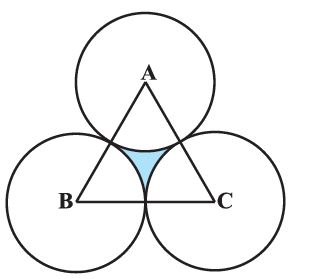### Solution

What is known?

(i) Area of an equilateral triangle $$\Delta {ABC} = 17320.5\,{\text{c}}{{\text{m}}^2}$$

(ii) with each vertex of a $$\Delta$$ as center a circle is drawn with radius

\begin{align} = \frac{1}{2}({\text{ length of side of }}\Delta {ABC})\end{align}

(Use $$\pi = 3.14$$ and $$\sqrt 3 = 1.73205$$)

What is unknown?

Reasoning:

(i) Since area of triangle is given , we can find side of $$\Delta {ABC}$$ using the formula of area of equilateral

\begin{align}\Delta = \frac{{\sqrt 3 }}{4}{({\text{side}})^2}\\{({\text{side}})^2} = \frac{{{\text{area}} \times 4}}{{\sqrt 3 }}\end{align}

(ii) Since radius\begin{align} {(r)} = \frac{1}{2} \end{align}( length of side of $$\Delta$$ ) we can find  $$\rm{}r$$

Also, all angles of an equilateral triangle are equal.

$$\therefore \;$$ Angle subtended by each sector \begin{align}(\theta ) = \frac{{{{180}^0}}}{3} = {60^\circ }\end{align}

Using the formula

Area of the sector of angle \begin{align} \theta = \frac{\theta }{{{{360}^ \circ }}} \times \pi {r^2}\end{align}

We can find Area of each sector \begin{align} = \frac{{60}}{{360}} \times \pi {r^2}\, = \frac{\pi }{6}{r^2}\end{align}

All sectors are equal as they have same radius $${r}$$ and \begin{align}{ \theta = 6}{{\text{0}}^{{o}}}\end{align}

(iii) Visually from the figure it is clear that:

Area of the shaded region $$=$$  Area of $$\Delta {ABC} - 3\; \times$$ Area of each sector

\begin{align}&= 17320.5 - 3 \times \frac{{\pi {r^2}}}{6}\\ &= 17320.5 - \frac{{\pi {r^2}}}{2}\end{align}

which can be easily solved using $$\pi = 3.14$$ (as given) and we already know $$\rm{}r$$

Steps:

(i) Area of equilateral $$\Delta = 17320.5\,{\text{c}}{{\text{m}}^2}$$

\begin{align}\frac{{\sqrt 3 }}{4}{({\text{side}})^2} &= 17320.5\,\,{\text{c}}{{\text{m}}^2}\\{\left( {{\text{side}}} \right)^2} &= \frac{{17320.5 \times 4}}{{\sqrt 3 }}\\&= { \frac{{17320.5 \times 4}}{{1.73205}}}\\&{\rm side = \sqrt {10000 \times 4} }\\&= { 100 \times 2}\\& ={ 200\,\,{\text{cm}}}\end{align}

$$\text{radius of each sector(r)}$$\begin{align}&= \frac{1}{2}\left( \rm{side} \right) \\&= \frac{1}{2}\left( {200} \right)\,{\text{cm}}\\ &= 100\,{\text{cm}}\end{align}

All interior angles of an equilateral $$\Delta$$ are of measure $${60^ \circ }$$

And all $$3$$ sectors are made using these interior angles.

$$\therefore\;$$ Angles subtended at the center by each sector $$(\theta) = {60^ \circ }$$

\begin{align}\text{Area of each sector}= \frac{{\rm{\theta }}}{{{{360}^ \circ }}} \times \pi {r^2}\end{align}

$$\text{Area of 3 sectors}$$\begin{align} &= 3 \times \frac{{{{60}^ \circ }}}{{{{360}^ \circ }}} \times \pi {r^2}\\&= 3 \times \frac{1}{6} \times 3.14 \times {\left( {100\rm{}\,cm} \right)^2}\\&= 15700\rm{}\,cm^2 \end{align}

Area of shaded region $$=$$ Area of Quadrant $$\rm{}\Delta{ABC}\,-$$ Area of 3 sector

\begin{align}&= 17320.5c{m^2} -15700c{m^2}\\&= 1620.5c{m^2}\end{align}

## Chapter 12 Ex.12.3 Question 11

On a square handkerchief, nine circular designs each of radius $$7\, \rm{cm}$$ are made (see Figure). Find the area of the remaining portion of the handkerchief.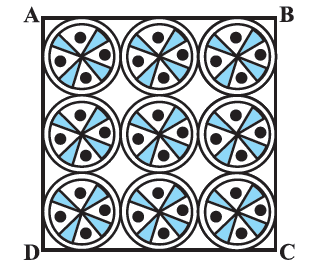### Solution

What is known?

On a square handkerchief, $$9$$ circular designs each of radius $${(r)} = \rm7cm$$ are made.

What is unknown?

Area of the remaining portion of the handkerchief.

Reasoning:

Radius of circular design $$\text{= 7 cm}$$

$$\therefore \;$$Diameter each circular design $$=\,7 \times 2 = 14{\text{cm}}$$

Visually and logically since all the $$3$$ circular design are touching each other and cover the entire length of the square.

$$\therefore \;$$Side of the square(s)= three times diameter of circular design

\begin{align}= 3 \times 14cm = 42cm\end{align}

Also, visually from the figure it is clear that:

Area of the remaining portion of handkerchief  $$=$$  Area of square $$- \,\,9 \times$$ (Area of each circular design)

$= {s^2} - 9\left( {\pi {r^2}} \right)$

Which can be easily solved since side $$(s)$$ of square and radius $${(r)}$$ are known.

Steps:

Radius of each circular design $$\rm{}r = 7\; \rm{cm}$$

$$\because\;$$ Diameter of each circular design $$\rm{}= 2r =2\times 7\,cm=\text{ 14 cm}$$

From the figure, it is observed that

Side of the square \begin{align}s = 3 \times 14cm = 42cm\end{align}

\begin{align}&= 14 \,\rm{cm}\, + 14 cm + 14 \,\rm{cm}\\ &= 42\,\rm{cm}\end{align}

Area of the remaining portion of the handkerchief = Area of square $$- \,9\; \times$$  (Area of each circular design)

\begin{align} &={{s^2} - 9\left( {\pi {r^2}} \right)}\\ &= {{{(42)}^2} - 9 \times \frac{{22}}{7} \times {{(7)}^2}}\\&= {1764 - 9 \times 22 \times 7}\\ &= {1764 - 1386}\\&= {378\,\,{\text{c}}{{\text{m}}^2}}\end{align}

## Chapter 12 Ex.12.3 Question 12

In Figure, $${OACB}$$ is a quadrant of a circle with centre $${O}$$ and radius $$3.5\; \rm{cm.}$$ If $$OD = 2 \,\rm{cm,}$$ find the area of the

(i) Quadrant $${OACB,}$$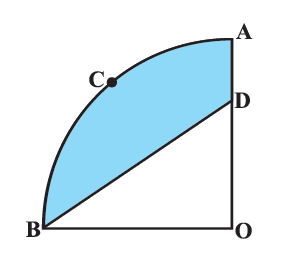### Solution

What is known?

$${OACB}$$ is a quadrant of a circle with centre $${O}$$ and radius $$(r) = \rm{3.5 cm}$$

What is unknown?

(i) Area of the quadrant $${OACB}$$

(ii) Area of the shaded region

Reasoning:

(i) Since quadrant mean \begin{align}\frac{1}{4}\rm{th}\end{align} part.

Therefore  angle  at the centre of a quadrant of a circle\begin{align} = \frac{{360}}{4} = {90^ \circ }\end{align}

Area of the quadrant OACB \begin{align} = \frac{1}{4}\pi {r^2}\end{align}

We can get area of quadrant $${OACB}$$ with radius  $$r = \text{3.5 cm}$$

(ii) Visually from the figure it is clear that

Area of shaded region $$=$$ Area of quadrant $$OACB\; –$$ Area of $$\Delta {BDO}$$

Since $$\angle {BOD} = {90^ \circ }$$

$$\therefore\;$$ For side OB of $$\Delta {BDO, OD}$$  is the

Using formula Area of triangle \begin{align} = \frac{1}{2} \times {\text{base}} \times {\text{height}}\end{align}

We can find Area of $$\Delta {BDO}$$ with base $$= OB = \text{3.5 cm}$$ (radius of quadrant) height $$= OD = \text{2 cm}$$

Steps:

(i)  Since $${OACB}$$ is a quadrant, it will subtend \begin{align} \text{} \,\, \theta = \frac{{{{360}^\circ }}}{4} = {90^\circ }\end{align} angle at $${O}$$

Radius $$(r) = \text{3.5 cm}$$

Area of quadrant $${OACB}$$ \begin{align}= \frac{1}{4}\pi {r^2}\end{align}

\begin{align}&= \frac{1}{4} \times \frac{{22}}{7} \times {\left( {3.5cm} \right)^2}\\&= \frac{1}{4} \times \frac{{22}}{7} \times \frac{7}{2} \times \frac{7}{2}c{m^2}\\&= \frac{{77}}{8}c{m^2}\end{align}

In

\begin{align}\Delta BDO,OB &= r = 3.5cm = \frac{7}{2}cm\\OD &= 2cm\,\angle BOD = {90^{\rm{\circ}}}\end{align}

\begin{align}{\rm{Area of }}\Delta BDO &= \frac{1}{2} \times {\rm{ }}base{\rm{ }} \times height\\&= \frac{1}{2} \times OB \times OD\\& = \frac{1}{2} \times \frac{7}{2}cm \times 2cm\\&= \frac{7}{2}c{m^2}\end{align}

From figure, it is observed that:

Area of shaded region $$=$$ Area of Quadrant $${OACB} \,-$$ Area of $$\Delta {BDO}$$

\begin{align}&= \frac{{77}}{8} - 3.5\,{\text{c}}{{\text{m}}^2}\\&={ \frac{{77}}{8} - \frac{7}{2}{\text{c}}{{\text{m}}^2}}\\&= {\frac{{77 - 28}}{8}{\text{c}}{{\text{m}}^2}}\\&= {\frac{{49}}{8}{\text{c}}{{\text{m}}^2}}\end{align}

## Chapter 12 Ex.12.3 Question 13

In Figure, a square $${OABC}$$ is inscribed in a quadrant $${OPBQ.}$$ If $$OA = \text {20 cm,}$$ find the area of the shaded region.

(Use $$\pi =3.14$$ )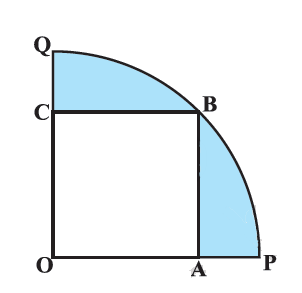### Solution

What is known?

A square $${OABC}$$ is inscribed in a quadrant $$OPBQ, OA =\text{ 20 cm.}$$

What is unknown?

Reasoning:

Visually from the figure it is clear that

Area of the shaded region $$=$$ Area of quadrant $${OPBQ}\, -$$ Area of square $${OABC}$$

Since side of the square $$OA =\text{ 20 cm}$$

$$\rm{OB =}$$ Radius $$=$$ Diagonal of the square $${OABC}$$

Using formula for

Area of sector of angle \begin{align}\theta = \frac{\theta }{{{{360}^\circ }}} \times \pi {r^2}\end{align}

With $$\theta = 90^\circ$$ and $$r = OB$$

We can find the area of the quadrant $${OPBQ.}$$

Steps: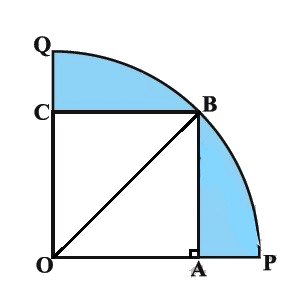Join $${OB.}$$

We know $$\Delta {OBA}$$ is a right angaled triangle, a $$\angle {OAB} \,= {90^ \circ }$$ (angle of a square)

$$\therefore\;$$Using Pythagoras theorem

\begin{align} \therefore\quad {{OB}^{2}}&={{OA}^{2}}+A{{B}^{2}} \\ &={{(20)}^{2}}+{{(20)}^{2}} \\ {DB}&=\sqrt{2{{(20)}^{2}}} \\ &=20\sqrt{2}\,\rm{cm}\end{align}

Therefore,radius of the quadrant,\begin{align}r = OB = 20\sqrt 2 cm\end{align}

\begin{align}&= \frac{{{{90}^\circ }}}{{{{360}^\circ }}} \times \pi {r^2}\\ &= \frac{1}{4} \times 3.14 \times {(20\sqrt 2 )^2}\\ &= \frac{1}{4} \times 3.14 \times {400} \times 2\\&= 628{\text{ cm}^2}\end{align}

Area of square OABC

\begin{align} &= {\left( {side} \right)^2}\\&= {\left( {OA} \right)^2}\\&= {\left( {20cm} \right)^2}\\&= 400c{m^2}\end{align}

Area of shaded region $$=$$ Area of quadrant $${OPBQ}\;-$$ Area of square $${OABC}$$

\begin{align}&= {628 - 400{\text{ cm}^2}}\\&= {228{\text{ cm}^2}}\end{align}

## Chapter 12 Ex.12.3 Question 14

$${AB}$$ and $${CD}$$ are respectively arcs of two concentric circles of radii $$\text{21 cm}$$ and $$\text{7 cm}$$ and center $${O}$$ (see Figure). If $$\angle {AOB} = 30^\circ$$, find the area of the shaded region.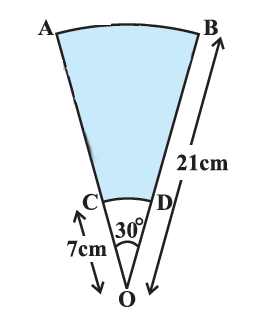### Solution

What is known?

$${AB}$$ and $${CD}$$ are arcs of two concentric circle of radii $$\text{21 cm}$$ and $$\text{7 cm}$$ respectively and centre $${O.}$$

$$\angle {{ AOB }}= \,30^\circ$$

What is unknown?

Reasoning:

Area of the shaded region $$=$$ Area of sector $${ABO}\, -$$ Area of sector $${CDO}$$

Areas of sectors $${ABO}$$ and $${CDO}$$ can be found by using the formula of

Area of sector of angle \begin{align} \theta = \frac{\theta }{{{{360}^\circ }}} \times \pi {r^2}\end{align}

where $${r}$$ is radius of the circle and angle.

with degree measure $$\theta$$

with $$\theta = {30^ \circ }$$ for the both the sector $${ABO}$$ and $${CDO}$$ angle $$\theta = {30^\circ}$$ and radii 21cm and 7cm respectively.

Steps:

Radius of the sector ABO,$$\rm{}R = OB = 21\rm{}cm$$

Radius of the sector CDO,$$\rm{}r = OD = 7\rm{}cm$$

For both the sector ABOand CDO angle,$$\theta = {90^\circ}$$

Area of shaded region $$=$$ Area of sector $${ABO}\; –$$ Area of sector $${CDO}$$

\begin{align}&= \frac{\rm{\theta }}{{360}^{\rm{o}}} \times \pi {R^2} - \frac{\rm{\theta}}{{360}^\circ } \times \pi {r^2}\\&= \frac{{\rm{\theta }}}{{{{360}^{\rm{o}}}}} \times \pi \left( {{R^2} - {r^2}} \right)\\ &= \frac{{{{30}^{\rm{o}}}}}{{{{360}^{\rm{o}}}}} \times \frac{{22}}{7}\left( {{{\left( {21cm} \right)}^2} - {{\left( {7cm} \right)}^2}} \right)\\&= \frac{1}{{12}} \times \frac{{22}}{7} \times \left( {441c{m^2} - 49c{m^2}} \right)\\&= \frac{{11}}{{42}} \times 392c{m^2}\\&= \frac{{308}}{3}c{m^2}\end{align}

## Chapter 12 Ex.12.3 Question 15

In Figure, $${ABC}$$ is a quadrant of a circle of radius $$14 \,\rm{cm}$$ and a semicircle is drawn with $${BC}$$ as diameter. Find the area of the shaded region.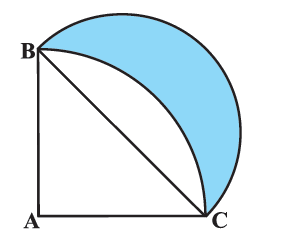### Solution

What is known?

$$ABC$$ is a quadrant of a circle of radius $$\text{14 cm}$$ and a semicircle is drawn with $${BC}$$ as diameter.

What is unknown?

Reasoning:

To visualize the shaded region better mark point $$D$$ on arc $${BC}$$ of quadrant $${ABC}$$ and $$E$$ on semicircle drawn with $${BC}$$ as diameter.

From the figure it is clear that

Area of the shaded region $$=$$ Area of semicircle $${BEC}\, -$$ Area of segment $${BDC}$$

•  To find area of semicircle $${BEC},$$ we need to find radius or diameter$$(BC)$$ of the semicircle.

$$\Delta BAC$$ is a right angled $$\Delta$$, right angled at $${A}$$ ($${ABC}$$ being a quadrant)

Using Pythagoras theorem, we can find the hypotenuse ($${BC}$$)

• To find area of segment $$BDC$$

Area of segment $${BDC}\;=$$  Area of quadrant $${ABC} \,-$$ Area of $$\Delta \, {ABC}$$

Area of quadrant $${ABC}$$ can be found by using the formula

Area of sector of angle \begin{align}\theta = \frac{\theta }{{{{360}^\circ }}} \times \pi {r^2}\end{align}

$${\text{Area of }}\Delta ABC$$\begin{align}&= \frac{1}{2} \times {\rm{ base }} \times {\rm{ height }}\\&= \frac{1}{2} \times AC \times AB\left( {\angle A = {{90}^\circ }} \right)\end{align}

Steps:

$$\Delta {ABC}$$ is a right angled $$\Delta$$, right angled at $${A}$$

\begin{align} {BC^2} &= {AB^2} = {AC^2}\\ &= {14\rm{cm}^2} + {14\rm{cm}^2}\\ BC &= \sqrt {2 + {{14}^2}} \\ &= 14\sqrt 2 {\text{ cm}}\end{align}

$$\therefore\;$$Radius of semicircle $$BEC$$,\begin{align}r = \frac{BC}{2} = \frac{14\sqrt 2 }{2}\rm cm = 7\sqrt 2 cm\end{align}

Area of the shaded region= Area of semicircle $$BEC$$ - Area of segment $$BDC$$

Area of shaded region $$=$$  Area of semicircle $${BEC} \,–$$ (Area of quadrant  $${ABDC}\;-$$ Area $$\Delta {ABC}$$)

\begin{align}&= \frac{{\pi {r^2}}}{2} \!\!-\!\! \left( \frac{{90}}{{360}} \!\times\! \pi {{\left( {14} \right)}^2} \! -\! \frac{1}{2} \!\times\! AC \!\times\! AB \right) \\&= \frac{{\pi {{\left( \!{7\sqrt 2 } \right)}^2}}}{2} \!-\! \left(\frac{{{{\left( {14} \right)}^2}\pi }}{4} \!-\! \frac{1}{2}\! \times \!14 \!\times \!14\! \right)\\&= \frac{{22\! \times\! 7 \!\times\! 7 \times 2}}{{7 \times 2}}\!\! -\! \! \left( \! \frac{{22 \!\times\! 14 \!\times\! 14}}{{7 \!\times \!4}} \!-\! 7 \!\times \!14 \!\right) \\&= 154 - \left( {154 - 98} \right)\\&= 98 \rm{cm^2}\end{align}

## Chapter 12 Ex.12.3 Question 16

Calculate the area of the designed region in Figure common between the two quadrants of circles of radius $$\text{8 cm}$$ each.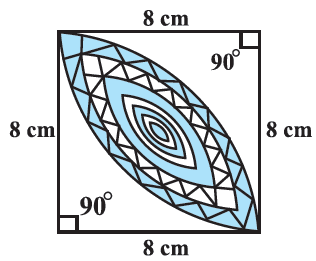### Solution

What is  known?

Designed region is the common area between the $$2$$ quadrants of circles of radius $$\text{8 cm.}$$

What is unknown?

Area of the designed region.

Reasoning:

In a circle with radius $$r$$ and angle at the center with degree measure $$\theta$$;

(i) Area of the sector \begin{align} = \frac{{\rm{\theta }}}{{{{360}^{\rm{\circ}}}}} \times \pi {r^2}\end{align}

(ii) Area of the segment = Area of the sector - Area of the corresponding triangle

From the figure,it is observed that

Area of the designed region = $$2\times$$Area of the segment of the quadrant of radius $$\rm8\,cm$$

$$2\times$$[Area of the quadrant - Area of the right triangle]

\begin{align} = \frac{{{{90}^ \circ }}}{{{{360}^ \circ }}} \times \pi {r^2} = \frac{1}{4}\pi {r^2}\end{align}

Area of the right triangle

\begin{align} = \frac{1}{2} \times \text{base} \times \text{height} \end{align}

Steps:

From the figure, it is observed that

Area of the designed region $$=2\times$$Area of the segment of the quadrant of radius $$\rm{}8\,cm$$

$$=2\times$$[Area of the quadrant - Area of the right triangle]

\begin{align}&= \!2\! \times\! \left[ \frac{1}{4}\pi {r^2} \!- \!\frac{1}{2} \!\times\! \text{base}\! \times\! \text{height} \right] \\&= \!2 \!\times\! \left[ \frac{1}{4} \!\times\! \frac{{22}}{7} \!\times\! 8 \rm cm \!\times\! 8cm \! - \!\frac{1}{2} \!\times\! \rm 8cm \!\times\! 8 \rm cm \right] \\&= \!2 \!\times\! \left[ {\frac{{352}}{7} \rm c{m^2} \!- \!32c{m^2}} \right]\\&= \!2 \!\times\! \left[ {\frac{{352 \!-\! 224}}{7} \rm c{m^2}} \right]\\&=\! 2 \times \frac{{128}}{7} \rm c{m^2}\\&= \frac{{256}}{7} \rm c{m^2}\end{align}